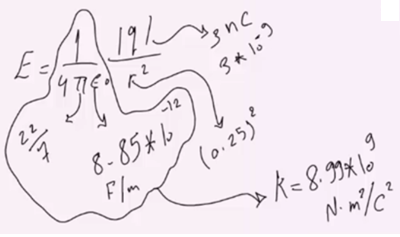Need Help?

Subscribe to Physics 2

###### \${selected_topic_name}
• Notes

A particle has charge $-3.00 \mathrm{nC}$ . (a) Find the magnitude and direction of the electric field due to this particle at a point
0.250m directly above it. (b) At what distance from this particle does its electric field have a magnitude of 12.0 N/C?

$q=-3nC$

$r=0.25 \mathrm{m}$$E=432 \mathrm{N/C}$

$r=? \quad (a) E=12 N / C$

$E=K \frac{|q|}{r^{2}} \Rightarrow r=\sqrt{\frac{k|q|}{E}}$

$r=\sqrt{\frac{8 \cdot 99 * 10^{9}+3 * 10^{-9}}{12}}=1.5m$